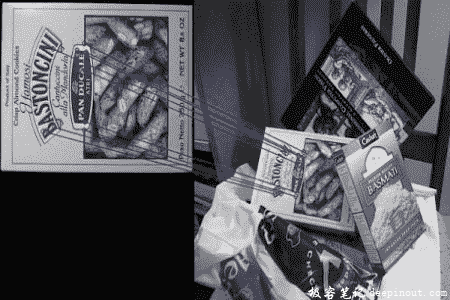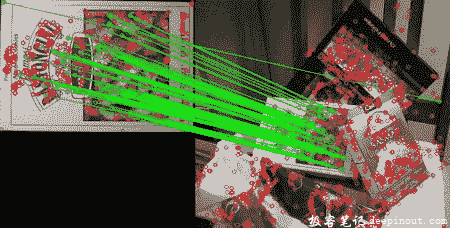# OpenCV-Python特征匹配

• 我们将看到如何将一个图像中的特征点与其他图像进行匹配。
• 我们将在 OpenCV 中使用蛮力（Brute-Force）匹配和 FLANN 匹配

## 蛮力匹配的基础知识

### 对 ORB 描述子使用蛮力匹配

import numpy as np
import cv2 as cv
import matplotlib.pyplot as plt
# Initiate ORB detector
orb = cv.ORB_create()
# find the keypoints and descriptors with ORB
kp1, des1 = orb.detectAndCompute(img1,None)
kp2, des2 = orb.detectAndCompute(img2,None)


# create BFMatcher object
bf = cv.BFMatcher(cv.NORM_HAMMING, crossCheck=True)
# Match descriptors.
matches = bf.match(des1,des2)
# Sort them in the order of their distance.
matches = sorted(matches, key = lambda x:x.distance)
# Draw first 10 matches.
img3 = cv.drawMatches(img1,kp1,img2,kp2,matches[:10], None, flags=2)
plt.imshow(img3),plt.show()### 这个匹配器对象是什么？

matches = bf.match(des1,des2)的结果是 DMatch 对象的列表。此 DMatch 对象具有以下属性：

• DMatch.distance – 描述子之间的距离。越低越好。
• DMatch.trainIdx – 目标图像中描述子的索引
• DMatch.queryIdx – 查询图像中描述子的索引
• DMatch.imgIdx – 目标图像的索引。

### 对 SIFT 描述符进行蛮力匹配和比率测试

import numpy as np
import cv2 as cv
from matplotlib import pyplot as plt
# Initiate SIFT detector
sift = cv.SIFT()
# find the keypoints and descriptors with SIFT
kp1, des1 = sift.detectAndCompute(img1,None)
kp2, des2 = sift.detectAndCompute(img2,None)
# BFMatcher with default params
bf = cv.BFMatcher()
matches = bf.knnMatch(des1,des2, k=2)
# Apply ratio test
good = []
for m,n in matches:
if m.distance < 0.75*n.distance:
good.append([m])
# cv.drawMatchesKnn expects list of lists as matches.
img3 = cv.drawMatchesKnn(img1,kp1,img2,kp2,good,flags=2)
plt.imshow(img3),plt.show()## 基于 FLANN 的匹配器

FLANN 代表快速最近邻搜索包（Fast Library for Approximate Nearest Neighbors）。它包含一系列算法，这些算法针对大型数据集中的快速最近邻搜索和高维特征进行了优化。对于大型数据集，它比 BFMatcher 工作得更快。我们将看到基于 FLANN 的匹配器的第二个示例。

index_params = dict(algorithm = FLANN_INDEX_KDTREE, trees = 5)


index_params= dict(algorithm = FLANN_INDEX_LSH,
table_number = 6, # 12
key_size = 12,     # 20
multi_probe_level = 1) #2


import numpy as np
import cv2 as cv
from matplotlib import pyplot as plt
# Initiate SIFT detector
sift = cv.SIFT()
# find the keypoints and descriptors with SIFT
kp1, des1 = sift.detectAndCompute(img1,None)
kp2, des2 = sift.detectAndCompute(img2,None)
# FLANN parameters
FLANN_INDEX_KDTREE = 1
index_params = dict(algorithm = FLANN_INDEX_KDTREE, trees = 5)
search_params = dict(checks=50)   # or pass empty dictionary
flann = cv.FlannBasedMatcher(index_params,search_params)
matches = flann.knnMatch(des1,des2,k=2)
# Need to draw only good matches, so create a mask
matchesMask = [[0,0] for i in xrange(len(matches))]
# ratio test as per Lowe's paper
for i,(m,n) in enumerate(matches):
if m.distance < 0.7*n.distance:
draw_params = dict(matchColor = (0,255,0),
singlePointColor = (255,0,0),
flags = 0)
img3 = cv.drawMatchesKnn(img1,kp1,img2,kp2,matches,None,**draw_params)
plt.imshow(img3,),plt.show()• 回顶